# How to count cells in Excel

Sometimes when you are working with big data, you need to know the number of cells in your data set, based on specific conditions. So in today’s tutorial we are going to learn how to count the number of cells in your data set having some key features.

In the data set shown above we’ll learn various ways to find out the number of cells in the data set based upon different conditions.

• Count cells with any data
• Count cells with blanks
• Count cells with text only
• Count cells with numeric data only
• Count cells with a specific word in it
• Count cells fulfilling a logical comparison condition

So let’s dive into the data and learn how we can use simple formulas to count the cells step by step.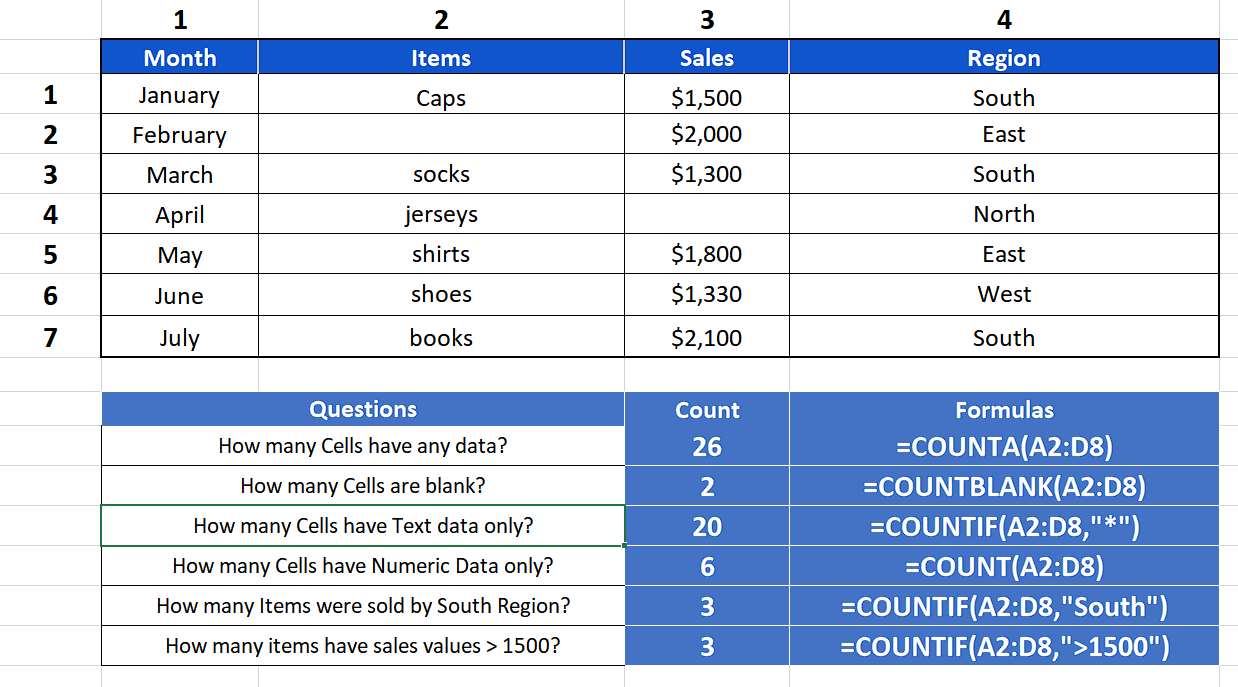Excel is a very powerful software when it comes to performing data analysis, simple mathematical, statistical or financial calculations. It comes up with a lot of functions which help us in doing the daily tasks.

### Step 1 – Count cells with any type of data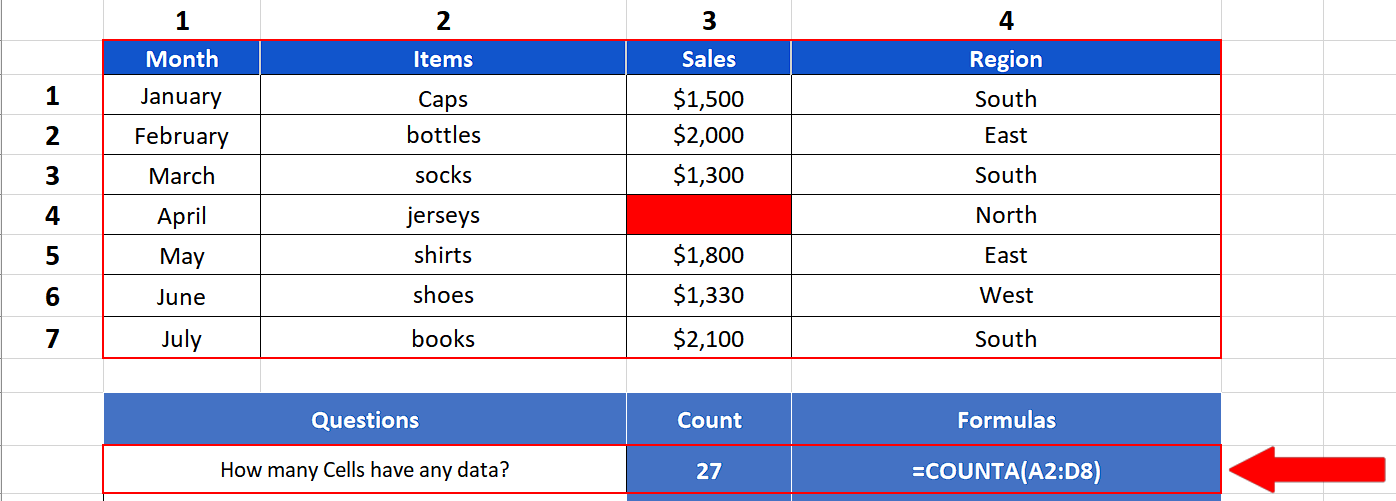– We can count the cells which have any type of data with a simple formula
=COUNTA(A2:D8)
COUNTA will count all those cells which have any data in it except the blanks. As we can see, our dataset had 28 cells in total. There was one blank cell in the data marked with red. So the result is 27 excluding the blanks.

### Step 2 – Count the number of blank cells in the data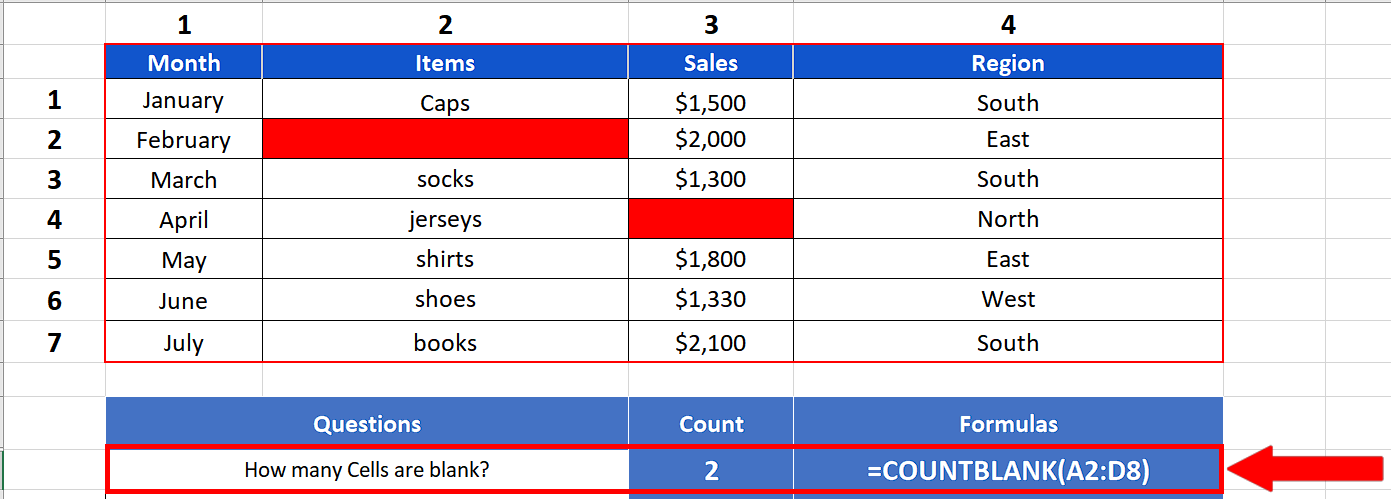– We can count the cells which have any type of data with a simple formula
=COUNTBLANK(A2:D8)
COUNTBLANK will count the cells only which are blank in the given range. As we have only two blanks, both cells marked with red, so the desired result is 2.

### Step 3 – Count the number of cells with text data only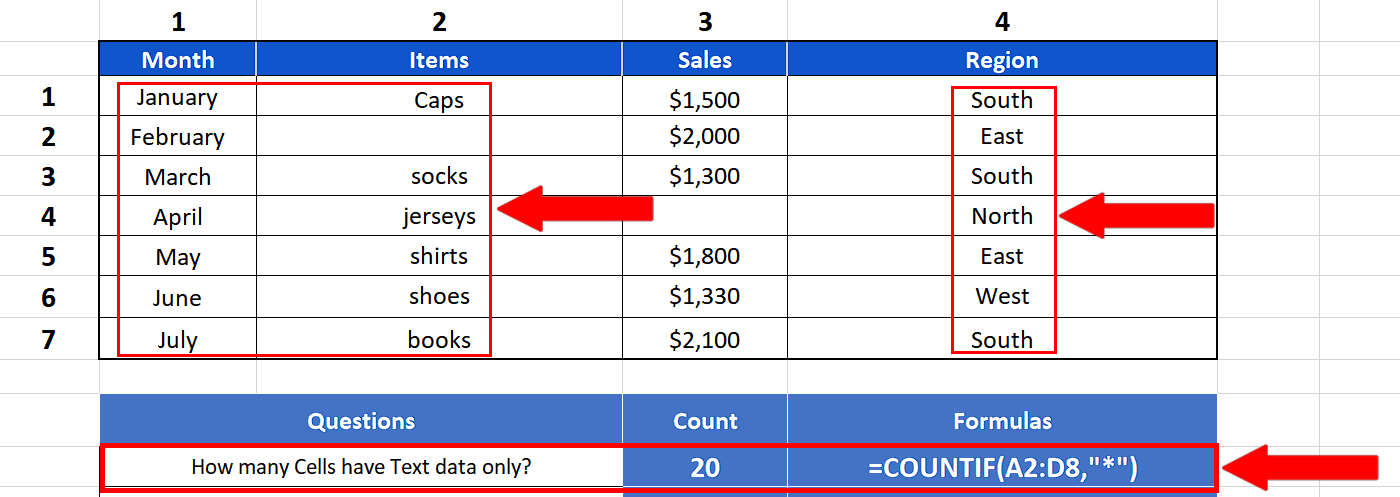– We can count the cells which have any type of data with a simple formula
=COUNTIF(A2:D8, “*”)
COUNTIF with wildcard “*” will count only those cells which have text data in it. As we can see, our dataset had numeric data and blank cells as well and only 20 cells contain text data, so the result is 20.

### Step 4 – Count number of cells with numeric data only– We can count the cells which have any type of data with a simple formula
=COUNT(A2:D8)
COUNT will count the cells only which have numeric data in them within the given range of data. As we have only six such cells so the desired result is 6.

### Step 5 – Count number of cells with specific text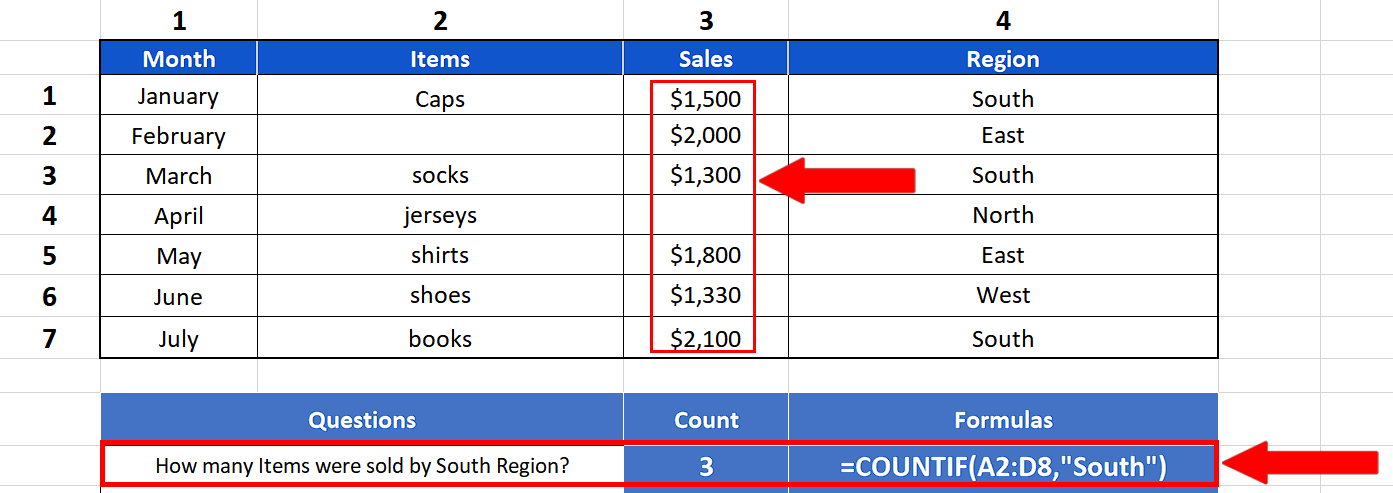– We can count the cells which have any specific text data with a simple formula
=COUNTIF(A2:D8, “South”)
COUNTIF with special keyword “South” will count only those cells which have the exact word “South” in it. This will give us the count of items sold by the South Region.

### Step 6 – Count cells meeting a logical condition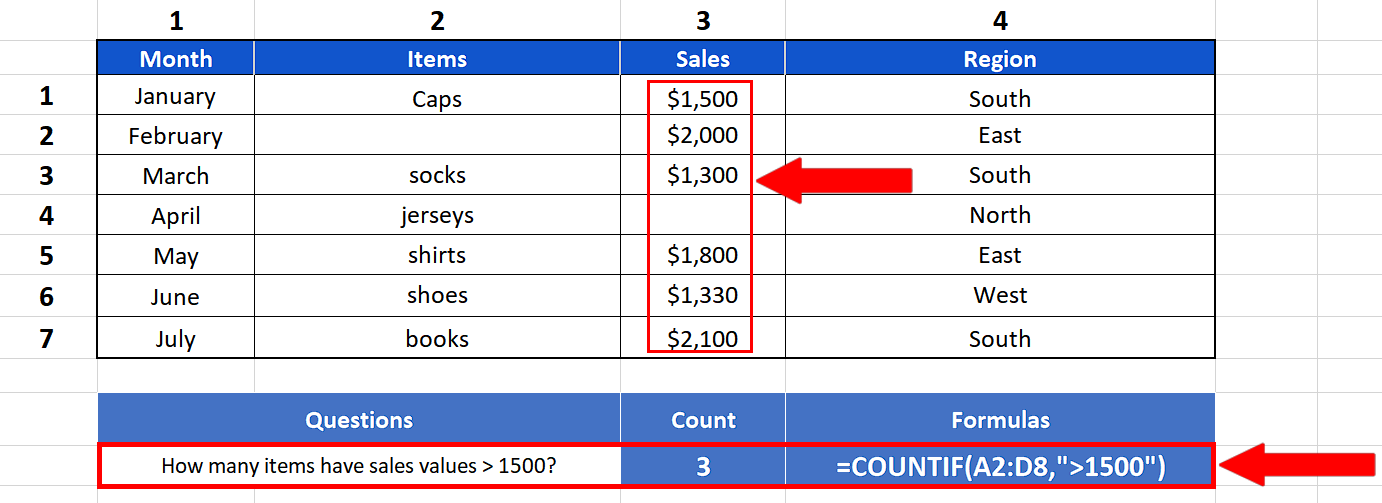– We can count the cells which have any type of data with a simple formula
=COUNTIF(A2:D8, “>1500”)
COUNTIF with special condition “>1500” will count the cells only which have sales values greater than 1500 within the given range of data and the desired result is 3.